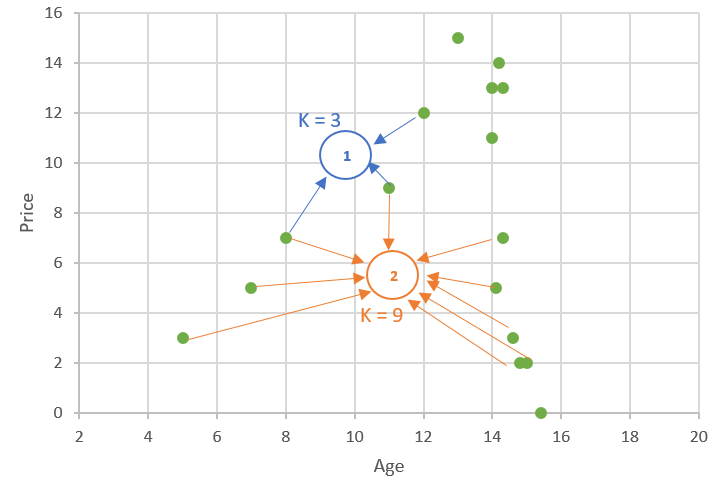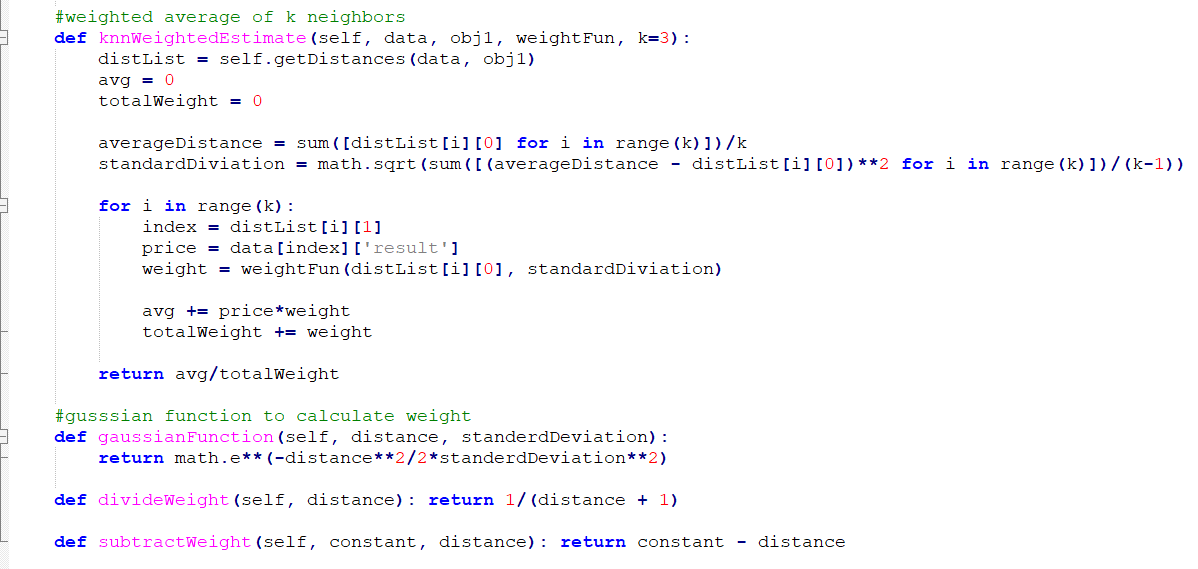# Estimate price of products using K-Nearest Neighbors (KNN), Implementation of K-Nearest Neighbors (KNN)

 Category: Machine Learning Tags:K-Nearest Neighbors Code Files

## Introduction

We have seen k-means clustering algorithm in previous article which creates k clusters of data. In this article, we will predict cost of products using k nearest neighbors. K-Nearest Neighbors algorithm searches K most similar products and based on that it calculates cost of a new product.

## Implementation

Suppose of a wine outlet, wine’s value will be depended on its age and rating. Wine’s value will increase until its peak age and once its peak age is crossed wine will expire soon and value will fall drastically. We will create a method to generate wine data of 200 bottles in class kNearestNeighbors.

```#generating random 200 wine data
def generateRandomWines(self):
wines = []
for i in range(200):
#rating in range of 0-5
rating = round(random.random()*5)
#age in range of 10-20
age = round(random.random()*10) + 10
price = self.winePrice(rating,age)
wines.append({'input':(rating,age), 'result':price})
return wines```

and winePrice method:

```#genearting price
def winePrice(self, rating, age):
peakAge = (rating+1)*20
price = (rating+1)
if(age > peakAge):
#after 5 years of peak age wine will be expired
price += price*(5 - (age - peakAge))
else:
#price will be increased in proportional to it's age
price += price*(5*(age+1)/peakAge)

if(price < 0): price = 0
return price```

generateRandomWines method returns 200 random wines with rating, age and price. Let's call above method and print top two wine data.

```knn = kNearestNeighbors()
wines = knn.generateRandomWines()
print(wines)
print(wines)```

Output:

{'input': (3.0, 14.0), 'result': 7.75}

{'input': (3.0, 16.0), 'result': 8.25}

We can see above input data has rating and age in input and result has price of wine. To find similarities in above two wines we can add Euclidean Distance method.

```#calculating eluclidean distance
def eluclideanDistance(self, obj1, obj2):
distance = math.sqrt(sum([(obj1[i] - obj2[i])**2 for i in range(len(obj1))]))
return distance```

We can calculate distance of top two wines like

```knn = kNearestNeighbors()
wines = knn.generateRandomWines()
print(knn.eluclideanDistance(wines['input'], wines['input']))```

Output:

5.0

Now we have wine data as well similarity function, remember that similarity is inversely proportional to distance, distance increases similarity decreases. Now we can implement k nearest neighbors method.

First, we must find distance score of all wines from input wine:

```#distance of object from all other training data
def getDistances(self, data, obj1):
distances  = []
for i in range(len(data)):
obj2 = data[i]['input']
#distance, index of data item
distances.append((self.eluclideanDistance(obj1, obj2), i))
distances.sort()
return distances```

Now we can simply calculate average of most similar k neighbors:

```def knnEstimate(self, data, obj1, k=3):
distList = self.getDistances(data, obj1)
avg = 0
#considering only k neighbors
for i in range(k):
index = distList[i]
avg += data[index]['result']

return avg/k```

Let’s calculate price of a new wine:

```knn = kNearestNeighbors()
#wine data
wines = knn.generateRandomWines()
#new wine which price to be calculated
newWine = (4,30)
print(knn.knnWeightedEstimate(wines, newWine, knn.gaussianFunction))```

Output:

9.91666666667

Above method calculates price of wine using average of k similar wines but this will not work well in some situations, see figure:Fig 1: K-Nearest Neighbors

You can see in figure above. Greens are data points, when we have k=3(small value of k) and for a new item 1 we will end up averaging neighbors which are not much similar, consider blue markup. If we take value of k=9(large value of k) and new item is 2 then we will end up underestimating it since most of the neighbors falls in low price, consider orange markup.

Now we have to choose value of k carefully, we can plot the data and choose it manually but it will not be efficient way of doing it. Other way is, instead of average we can make use of weighted average and weight can calculated on distance. High distance will lead to less weight and low distance will lead to high weight. We can create weight function using below methods:

1. Divide Method: We can divide 1 by distance but this will give around zero weight for high values of distance and as distance increase it will fall drastically.
`def divideWeight(self, distance): return 1/(distance + 1)`
2. Subtract Method: We can subtract distance from any constant, but here choosing constant is a problem.
`def subtractWeight(self, constant, distance): return constant - distance`
3. Gaussian method: In this for higher values of distance weight will not fall drastically if forms a bell graph which never touches zero for even very high values of distance.

We will use Gaussian method which formula is given below:

Let’s write Gaussian function in code and we will ignore constants from the function:

```#gusssian function to calculate weight
def gaussianFunction(self, distance, standerdDeviation):
return math.e**(-distance**2/2*standerdDeviation**2)```

Now we will create method knnWeightedEstimate

```    #weighted average of k neighbors
def knnWeightedEstimate(self, data, obj1, weightFun, k=3):
distList = self.getDistances(data, obj1)
avg = 0
totalWeight = 0

averageDistance = sum([distList[i] for i in range(k)])/k
standardDiviation = math.sqrt(sum([(averageDistance - distList[i])**2 for i in range(k)])/(k-1))

for i in range(k):
index = distList[i]
price = data[index]['result']
weight = weightFun(distList[i], standardDiviation)

avg += price*weight
totalWeight += weight

return avg/totalWeight```

Let’s run the code

```knn = kNearestNeighbors()
#wine data
wines = knn.generateRandomWines()
#new wine which price to be calculated
newWine = (4,30)
print(knn.knnWeightedEstimate(wines, newWine, knn.gaussianFunction))```

Output:

9.25

We have estimated wine prices using k nearest neighbors, there might be chances we may need to scale the data if one or more dimensions of data is having very small or very large value range. We can simply scale data by just multiplying all data in that dimension by some constant value.

 Like0 People
Last modified on 11 October 2018Nikhil Joshi Ceo & Founder at Dotnetlovers Atricles: 132 Questions: 9 Given Best Solutions: 9 *

### Reference:

programming collective intelligence - by Toby Segaran and published by O'Reilly

## Comments:Hi ,

Good article. Can you help me explain the weightfun being referenced in the code. Definition seems to be missing in the sample code. would you be kind to update the code files to include same.

Like1 Person on 12 October 2018Hi Abhijit,

Weight function can use divide method, subtract method or gaussian method internally to calculate weighted average of distances, suppose there are three neighbors so we have to consider price of these three with respect to their distances, if distance is low than impact on price should be high and if distance is high than price should be considered as low. I used only gaussian method because that is best way to calculate weighted average here. I can see all these functions are available in sample code:Weight functions for KNN
Like0 People on 12 October 2018Hi Nikhil ,

Thanks for response and explanation.

Like1 Person on 15 October 2018

You are not loggedin, please login or signup to add comments:

## Existing User

Login via:## New Userx